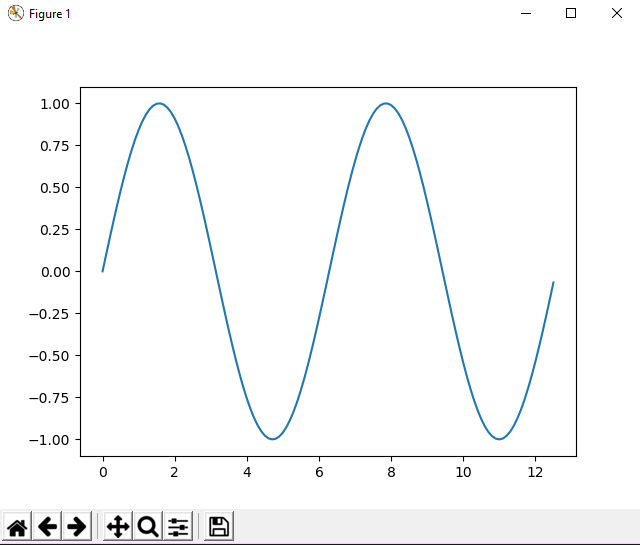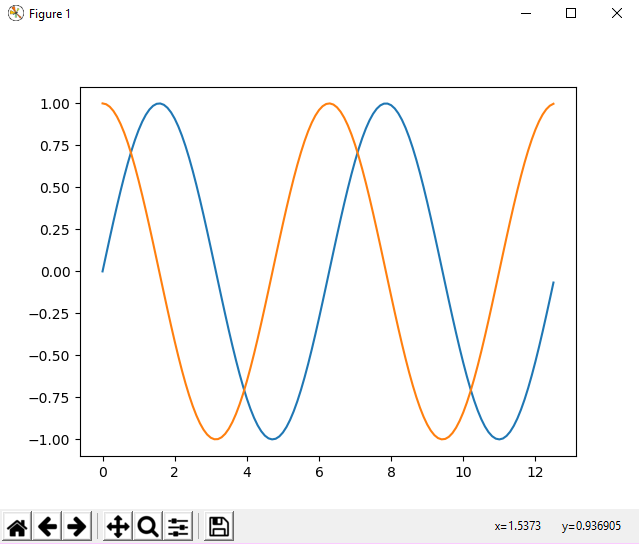# Plotting sine and cosine graph using matloplib in python

Plotting is an essential skill. Plots can reveal trends in data and outliers. Plots are a way to visually communicate results with your team and customers. In this tutorial, we are going to plot a sine and cosine functions using Python and matplotlib. Matplotlib is a plotting library that can produce line plots, bar graphs, histograms and many other types of plots using Python. Matplotlib is not included in the standard library. If you downloaded Python from python.org, you will need to install matplotlib and numpy with pip on the command line. for this refer

## Sine and Cosine Graph Using matplotlib in Python

In this tutorial, we are going to build a couple of plots which show the trig functions sine and cosine. We’ll start by importing matplotlib using the standard lines import matplotlib.pyplot as plt. This means we can use the short alias plt when we call these two libraries.

Import required libraries to draw sine and cosine graph in Python – matplotlib and numpy

```import matplotlib.pyplot as plt
import numpy as np```

Next, we will set an x value from zero to 4π in increments of 0.1 radians to use in our plot. The x-values are stored in a numpy array. has three arguments: startstopstep. We start at zero, stop at 4π and step by 0.1 radians. Then we define a variable `y` as the sine of x using numpy sine() function.

```x = np.arange(0,4*np.pi,0.1)   # start,stop,step
y = np.sin(x)```

To build the plot, we use matplotlib’s plt.show() function. The two arguments are our numpy arrays x and y . The syntax plt.show() will show the finished plot.

```plt.plot(x, y)
plt.show()```

Now we will build one more plot, a plot which shows the sine and cosine of x and also includes axis labels, a title, and a legend. We build the numpy arrays using the functions as before:

```x = np.arange(0,4*np.pi,0.1)   # start,stop,step
y = np.sin(x)
z = np.cos(x)```

now using plt.show().

```plt.plot(x,y,x,z)
plt.show()```

### Output of sine and cosine graph program in Python:

Here are the two screenshots of the output of the program:sine graph in matplotlib – pythoncosine graph in matplotlib – Python

This is how we have built our graph, so we have learned the following.

• what is matplotlib
• importing matplotlib
• plotting sine and cosine graph

hope you got a fair idea about matplotlib and graph relation.see you in the next tutorial until then enjoy learning.